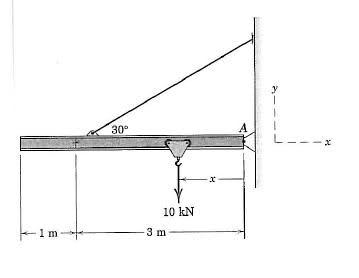# Statics: Varying Force

## Homework Statement

The jib crane is designed for a maximum capacity of 10kN, and its uniform I beam has a mass of 200kg.
a)Plot the magnitude R of the force on the pin A as a function of x through its operating range of x=0.2m to x=3.8m.
b)Determine the minimum value of R and the corresponding value of x## The Attempt at a Solution

Totally stumped on this one. It'll be great if someone could point me in the right direction.
I didn't even think the force would vary with distance :S. Isn't it only the moment about the point that changes? How do I determine the change in force with distance?

## Answers and Replies

minger
Science Advisor
The pin can only resist force in the y-direction due to the definition of a pin. This means that the resultant force at the pin will be the sum of all the forces in the y-direction. Obviously there is a constant -10kN due to the load, however the resultant force in the y-direction at the cable will be a function of the position.

Sum moments about the pin to get the vertical force in the cable. Then sum forces in the y-direction to get the force at the pin. Get three points to see if it's linear, if it is, then use the equation of a line.